# GMAT Math : Calculating the slope of a tangent line

## Example Questions

### Example Question #1 : Calculating The Slope Of A Tangent Line

Find the slope of the line tangent toat.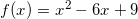Explanation:

f(x) in this case gives us a parabola. If we factor the f(x) we get: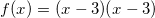This equation has one zero and that is at x=3. This also means we have a minimum at x=3.

The slope of a line tangent to a minimum or maximum is always 0, so our slope is zero.

### Example Question #2 : Calculating The Slope Of A Tangent Line

Calculate the slope of the tangent line to the following curve at the point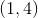.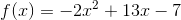Explanation:

The slope of the tangent line to a curve at any point is simply the slope of the curve at that point. To find the slope of the function at any point, we take the derivative: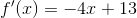Now we can plug in the x value of the given point,, which gives us the slope of the tangent line to the curve at that point: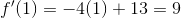### Example Question #3 : Calculating The Slope Of A Tangent Line

Calculate the slope of the line tangent to the curve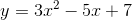at the point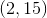.Explanation:

To calculate the slope of a tangent line at a particular point, we need to know the slope of the curve at that point. In order to find the slope of a curve at any point, we need to calculate its derivative: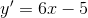The derivative describes the slope of the curve at any point, so we need to plug in thevalue of the given pointto find the slope of the tangent line at that location: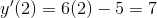### Example Question #4 : Calculating The Slope Of A Tangent Line

Calculate the slope of the line tangent to the following curve at: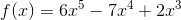Explanation:

First find the derivative of the function, and then plug in the given x coordinate, which will give the slope of the function at that location. The slope of the tangent line to a curve at a given location is equal to the slope of the function at that location: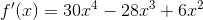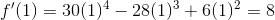### Example Question #5 : Calculating The Slope Of A Tangent Line

Calculate the slope of the line tangent to the following curve at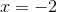: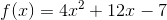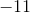Explanation:

First find the derivative of the function, and then plug in the given x coordinate, which will give the slope of the function at that location. The slope of the tangent line to a curve at a given location is equal to the slope of the function at that location: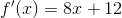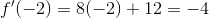### Example Question #6 : Calculating The Slope Of A Tangent Line

Calculate the slope of the line tangent to the following curve at: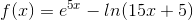Explanation:

First find the derivative of the function. The slope of the tangent line to a curve at a given point is equal to the slope of the function at that point, and the derivative of a function tells us its slope at any point. Plugging the given point into the equation for the derivative, we can calculate the slope of the function, and therefore the slope of the tangent line, at that point: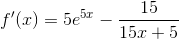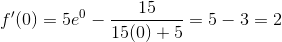Tired of practice problems?

Try live online GMAT prep today.